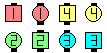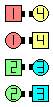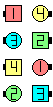Definitions of Square Dance Calls and Concepts

From Parallel Lines with the Ends Back-to-Back, or other applicable formations. EN: 10
Ends \$B\$,GXCf9g\$o\$;\$NJ?9T\$J(B Line, \$B\$^\$?\$OB>\$NE,@Z\$J(B formation \$B\$+\$i(B. JP: 10

Ends Bend and Turn Thru as Centers Face In and Cross Trail ThruEN: 20
Ends \$B\$O(B, Ends Bend, Turn Thru \$B\$r\$7(B, Centers \$B\$O(B, Face In, Cross Trail Thru \$B\$r\$7\$^\$9(B. JP: 20

Parallel Lines end in Back-to-Back Lines. Scramble is a 2-part call. EN: 30
\$BJ?9T\$J(B Line \$B\$+\$i\$O(B, \$BGXCf9g\$o\$;\$N(B Line \$B\$G=*\$o\$j\$^\$9(B. Scramble \$B\$O(B 2 \$B%Q!<%H\$N%3!<%k\$G\$9(B. JP: 30Scramble\$B\$NA0(B Ends Bend as Centers Face In(1/2)\$B\$N8e(B Ends Turn Thru as Centers Cross Trail Thru\$B\$N8e(B (\$B=*\$o\$j(B)